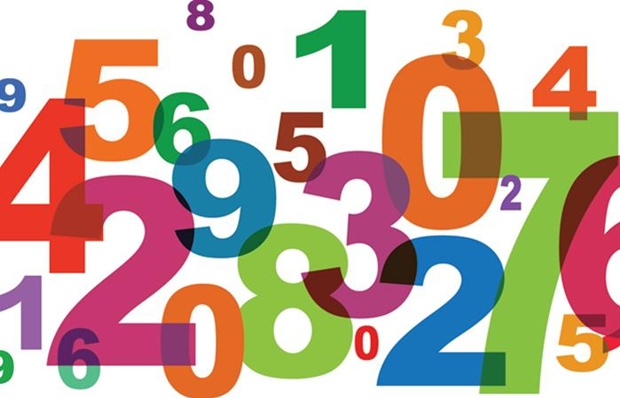# The RESULTANT.....

Algebra Level 2Tushar wrote a two digit number. He added 5 to the tens digit and subtracted 3 from the units digit of the number. The resulting number is twice the original number. Find the original number ?

×